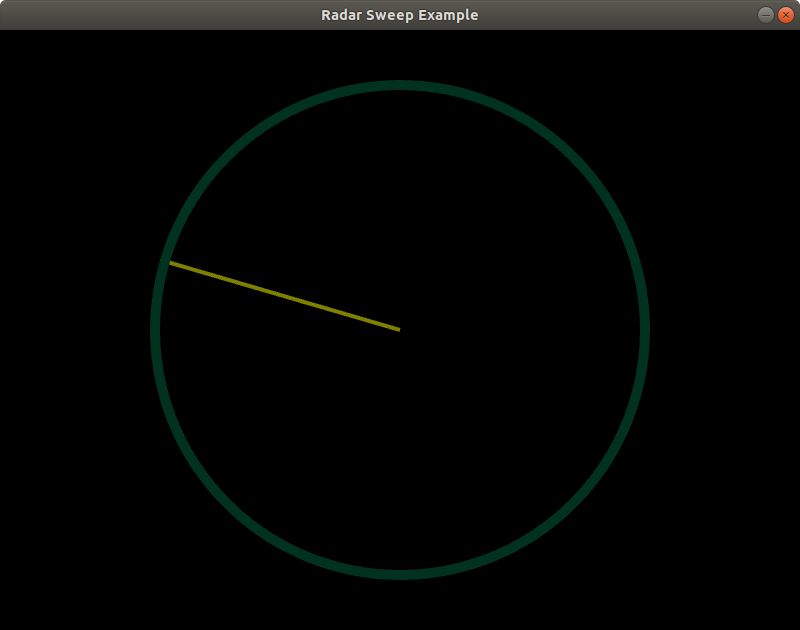Related Articles

• Difficulty Level : Expert
• Last Updated : 19 Oct, 2020

The Radar Sweep are used for displays of single level sweeps of radar data, and their display appears in the Main Display window. With the help of arcade module of Python, it is possible to perform a radar sweep animation. Before starting, it is highly recommended to revise concepts of arcade library.

Step 1: Import arcade as well math module in you respective Ide.

```import arcade
import math```

Step 2: Specify the parameters for the output window.

```# Set up the constants
WIDTH = 800
_HEIGHT = 600

```CENTER_X = SCREEN_WIDTH // 2
CENTER_Y = SCREEN_HEIGHT // 2
SWEEP_LENGTH = 250```

Step 4: Define a on_draw function, under which move the angle of the sweep and calculate the end point of our radar sweep, using math . Lastly draw the outline of the radar.

```def on_draw(_delta_time):
# Move the angle of the sweep.

# Calculate the end point of our radar sweep.
x = SWEEP_LENGTH * math.sin(on_draw.angle) + CENTER_X
y = SWEEP_LENGTH * math.cos(on_draw.angle) + CENTER_Y

# Start the render.

# Draw the outline of the radar

# This is a function-specific variable i.e
# we need to give them initial
# values.
on_draw.angle = 0  ```

Step 5: Define main function.

```def main():

# Open up our window
SCREEN_TITLE)

# Tell the computer to call the draw command at
# the specified interval.

# Run the program

#  close the window.
main()```

The radar sweep will looks like this –Complete source code:

## Python3

 `import` `arcade``import` `math`` ` `# Set up the constants``SCREEN_WIDTH ``=` `800``SCREEN_HEIGHT ``=` `600``SCREEN_TITLE ``=` `"Radar Sweep Example"`` ` `# These constants control the particulars ``# about the radar``CENTER_X ``=` `SCREEN_WIDTH ``/``/` `2``CENTER_Y ``=` `SCREEN_HEIGHT ``/``/` `2``RADIANS_PER_FRAME ``=` `0.02``SWEEP_LENGTH ``=` `250`` ` ` ` `def` `on_draw(_delta_time):`` ` `    ``# Move the angle of the sweep.``    ``on_draw.angle ``+``=` `RADIANS_PER_FRAME`` ` `    ``# Calculate the end point of our radar sweep. Using math.``    ``x ``=` `SWEEP_LENGTH ``*` `math.sin(on_draw.angle) ``+` `CENTER_X``    ``y ``=` `SWEEP_LENGTH ``*` `math.cos(on_draw.angle) ``+` `CENTER_Y`` ` `    ``# Start the render.``    ``arcade.start_render()`` ` `    ``# Draw the radar line``    ``arcade.draw_line(CENTER_X, CENTER_Y, x, y, arcade.color.OLIVE, ``4``)`` ` `    ``# Draw the outline of the radar``    ``arcade.draw_circle_outline(CENTER_X, CENTER_Y, SWEEP_LENGTH,``                               ``arcade.color.DARK_GREEN, ``10``)`` ` ` ` `on_draw.angle ``=` `0`` ` ` ` `def` `main():`` ` `    ``# Open up our window``    ``arcade.open_window(SCREEN_WIDTH, SCREEN_HEIGHT, SCREEN_TITLE)``    ``arcade.set_background_color(arcade.color.BLACK)`` ` `    ``# Tell the computer to call the draw command at the specified interval.``    ``arcade.schedule(on_draw, ``1` `/` `80``)`` ` `    ``# Run the program``    ``arcade.run()`` ` `    ``# close the window.``    ``arcade.close_window()`` ` `main()`

Output:

Attention geek! Strengthen your foundations with the Python Programming Foundation Course and learn the basics.

To begin with, your interview preparations Enhance your Data Structures concepts with the Python DS Course. And to begin with your Machine Learning Journey, join the Machine Learning – Basic Level Course

My Personal Notes arrow_drop_up